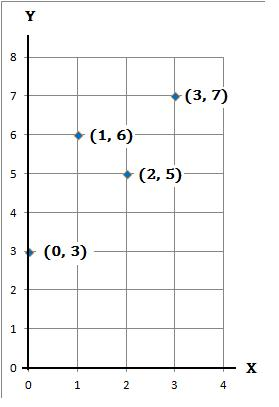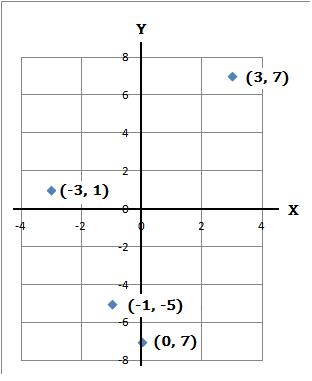### Coordinates

Coordinates are a set of numbers that shows a certain position on a graph or map. The first number is the horizontal position (either right or left) and the second number is the vertical the position (either up or down). The X axis represents the horizontal and the Y axis represents the vertical.

#### Example 1.   Plot the four coordinates (0, 3), (1, 6), (2, 5) and (3, 7) on a graph

(a) Make a table of the coordinates

 x 0 1 2 3 y 3 6 5 7

(b) Plot the coordinates#### Example 2.   Plot the four coordinates (−3, 1), (−1, −5), (0, −7) and (3, 7) on a graph

(a) Make a table of the coordinates

 x −3 −1 0 3 y 1 −5 −7 7

(b) Plot the coordinatesto: